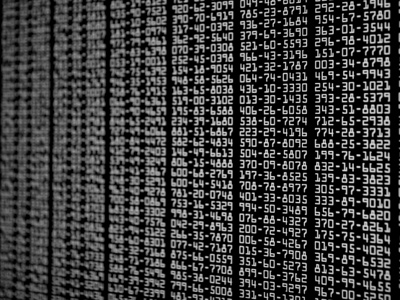If the range is large, the variation of the data is large.

# Unit 4 - Range

This GCSE Biology quiz is all about the range of data. In a scientific investigation, the range describes the maximum and minimum values of the independent or the dependent variables studied. It helps to detect any patterns in the data. Range is usually quoted as '10 mm to 70 mm' or '70 mm to 10 mm'. Top grade students would be using this to explain the limitations of their results, for example 'between 10 mm and 70 mm the pattern is ...'. They would then go on to say that it would be useful to conduct further work to investigate a higher and lower range.

In mathematics, the range of data is defined as the difference between the lowest number (minimum) and the highest number (maximum) in the data set and is useful when applied to the data obtained from repeating an experiment. The smaller the range of the repeats, the higher the precision of the experiment and the more reliable the data. Top grade candidates in science will be using mathematics to analyse their results. They could use the range to help their arguments for or against the reliability of their experiment.

Take for example these two sets of data obtained by different groups of students carrying out the same experiment:

Team A: 12, 12, 10.5, 10, 12, 10, 12.5

Team B: 14, 11.5, 12, 9, 12, 10.5, 10

Each team of students carried out the experiment 7 times and by pure chance, both sets of results give the same mean value. The range of the data of team A is 2 (their maximum value is 12.5 and their minimum 10.5) but the range for team B is 5 (their maximum value is 14 and their minimum 9) so you can say that the results of team A are more precise. If the two teams were to carry out the same experiment once more, team A would be more likely to be closer to the mean value.

1.
If the range is large, the variation of the data is...
large
small
insignificant
unchanged
The upper and lower limits are significantly different to each other and looking at possible reasons for a large spread of results could help you to suggest modifications to the experiment to increase reliability
2.
The range will guide us about a valid comparison between our experimental results and the control. If the ranges do not overlap, we say that there is...
no difference
a significant difference
a fluke
a chance that they may be different
If there is no overlap between the ranges, the experimental group must be different from the control and therefore we have a clear cut difference
3.
If the range is large, we need to consider this.
Repeating the experiment many more times
Leaving it and working out the average
Changing the experiment altogether
If the range is very wide, it could just be the method or it could be genuine biological variation. Repeating the experiment is the best way forward as a first step to double checking the data
4.
If there is a very wide range of data for the same experiment, we say that the data is...
precise
unreliable
ranging
inaccurate
Range is taken as a measure of reliability when applied to the repeat results of an experiment
5.
Which is the lower limit of this range of data?
22, 14, 16, 19, 11, 28
11
15
16
17
The lower limit is the smallest number
6.
To calculate the range, we...
subtract the largest number from the smallest
subtract the smallest number from the largest
subtract the mean from the largest number
take an average
This is a way of expressing the range as a single figure
7.
Which one of the following is the range of this data?
25, 16, 18, 10, 12, 16, 17
12 to 16
10 to 17
10 to 25
25 to 17
Remember, the range is the lowest to highest or vice-versa
8.
What is the upper limit of the following data?
15, 18, 25, 18, 45
15
18
25
45
The upper limit is the highest number
9.
The range is the same thing as this.
Average
Accuracy
Median
The range gives us a measure of the spread of the data
10.
Calculate the range of the following data.
12, 14, 16, 13, 17, 19
Six
Seven
Eight
Nine
The upper limit is 19, the lower limit is 12 so the range is 19 - 12 = 7